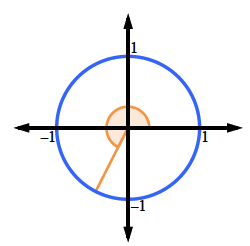### Home > INT3 > Chapter 9 > Lesson 9.1.6 > Problem9-77

9-77.

Calculate the value of each expression. Give an exact answer, if possible. Each angle measure is given in radians.

1. $\text{sin}\left(4\right)$

$−0.76$

1. $\text{sin}( \frac { 4 \pi } { 3 } )$

$\text{Since }\frac{4\pi}{3} \text{ is related to the }30^\circ-60^\circ-90^\circ\text{ angles, this one}$

$\text{has an exact answer. Locate it on the unit circle.}$

$\text{Notice that it will be a reflection (twice) of sin} \left(\frac{\pi}{3}\right).$

It is in the third quadrant.